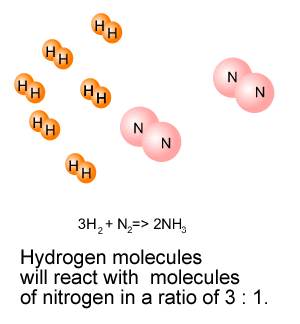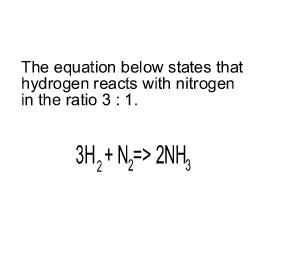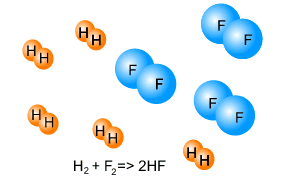Stoichiometry excess and limiting Chemical equations simply tell us in what ratio the reactants should be mixed in order to completely react. The formation of water from hydrogen and oxygen gases is given below. 2H2(g) + O2(g) => 2H2O(g) The equation states that hydrogen and oxygen molecules react in the ratio 2:1. So, 2 molecules of hydrogen will completely react with 1 molecule of oxygen. Complete reaction will also occur when: -12 molecules of oxygen react with 6 molecules of oxygen. -8 molecules of oxygen react with 4 molecules of oxygen. In fact, any combination of hydrogen and oxygen molecules, in the ratio 2:1 will react completely. Lets take the formation of ammonia (NH3) from hydrogen (H2) and nitrogen (N2) gases according to the equation below. 3H2(g) + N2(g) => 2NH3(g) Molecules of hydrogen react with molecules of nitrogen in the ratio 3:1. Are all the reactants completely used up if 6 molecules of hydrogen react with 2 molecules of nitrogen? Consider the animation on the right.What will happen if 6 molecules of hydrogen are mixed with 3 molecules of nitrogen? Consider the animation on the right. The reactants are not in the ratio 3:1. One of the reactants is left unreacted. We call this reactant the excess reagent. The reactant that is used up is known as the limiting reagent, because this will be totally used up and so stop the reaction from proceeding any further. In stopping the reaction it limits the amount of product formed, hence the name "limiting reagent" is used.Consider the image on the right. It shows hydrogen gas reacting with fluorine gas according to the equation given.   Identify the limiting and excess reagents. SolutionIn the above discussion we consider individual molecules. However, this is highly impractical and we use mol of substance instead. The way we deal with mol is the same as when we deal with individual molecules. For example Lets take the formation of ammonia (NH3) from hydrogen (H2) and nitrogen (N2) gases according to the equation below. 3H2(g) + N2(g) => 2NH3(g) Mol of hydrogen react with mol of nitrogen in the ratio 3:1. If 6 mol of hydrogen react with 3 mol of nitrogen then 1 mol of nitrogen is left unreacted.

Worksheet

Continue